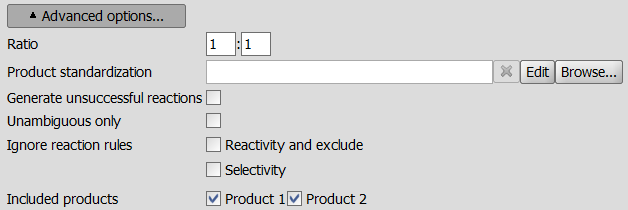# Ratio - Reactor GUIReactor Advanced options

Reactor GUI
Select the "Set Reactor Options" window, "Advanced options..." section to set reactant ratio different from that in the reaction equation.

Note that, the number and order of Ratio fields are in accordance with the number and order of reactants.

Ratio: Reactant ratio is considered as the number of cycles of the reaction. Zero (0) means an infinite number of cycles. This consideration is also feasible when only one reagent can be set in a reaction. For example, a reaction on a primary amine group will be repeated until all hydrogens are substituted if the ratio coefficient is 0 and will be stopped at a secondary amine if the coefficient is 1.

User's Guide

Reactor GUI

Reactor Home

User's Guide

Reactor CLI

Reactor Glossary